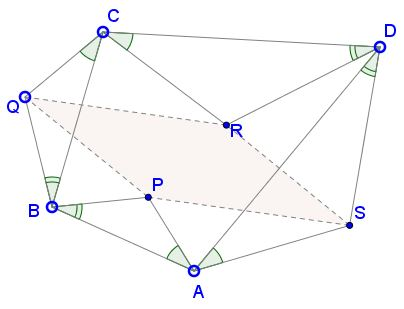# Similar Triangles on Sides of a QuadrilateralWhat is it about? A Mathematical Droodle

13 March, 2017, Created with GeoGebra

DiscussionThe applet may suggest the following statement [de Villiers]:

On the sides of an arbitrary quadrilateral ABCD similar triangles ABP, CBQ, CDR, ADS are constructed, so that the angles at the vertices P, Q, R, and S are equal, as are their angles sharing any of the vertices A, B, C, or D. Then the quadrilateral PQRS is a parallelogram.This clear generalization of a problem where all four triangles are equilateral was discovered by Michael de Villiers and confirmed with the dynamic geometry software. Having proved the theorem, he later discovered an earlier proof by R. Finney who made a reference to a 1881 result.

The proof follows that of a seemingly simpler problem with equilateral triangles, except that spiral similarities take place of the rotations.

Let (M, r, a) stand for the spiral similarity with center M, coefficient r, and angle a. The sum of any two spiral similarities is either a rotation, a translation, or a homothety. As a spiral similarity, homothety is given by (M, r, 0), which is a translation iff r = 1.

Assume the common angle at vertices P, Q, R, and S equals q. Consider the four spiral similarities: TP = (P, PB/PA, q), TQ = (Q, QC/QB, -q), TR = (R, RD/RC, q), and TS = (S, SA/SD, -q). The sum of the four is the spiral similarity with A as a fixed point and angle 0:

 (1) TP + TQ + TR + TS = (A, PB/PA·QC/QB·RD/DC·SA/SD, 0).

However, triangles ABP, CBQ, CDR, ADS:

 (2) PB/PA = QB/QC = RD/RC = SD/SA.

Thus the homothety coefficient in (1) equals

 (3) r = (PB/PA·QC/QB)·(RD/DC·SA/SD) = 1·1 = 1.

We see that the sum of the four transformations is a translation with a fixed point, i.e. the identity transformation. Along the way we also found that the sum of any two consecutive transformations is a genuine translation whose inverse is the sum of the other two transformations (executed in a certain order of course.)

Let P' be the image of P under the sum TP + TQ and R' be the image of R under TR + TS. Then triangles PQP' and RSR' are similar and similarly oriented. PP' and RR' are equal, parallel, but point in opposite directions. The same is true of other pairs of the corresponding sides, e.g. PQ and RS. The quadrilateral PQRS is therefore a parallelogram.

### References

1. M. de Villiers, The Role of Proof in Investigative, Computer-based Geometry: Some Personal Reflections, in Geometry Turned On, MAA Notes 41, 1997, pp. 15-24
2. R. L. Finney, Dynamic Proofs of Euclidean Theorems, Math Magazine, 43, pp. 177-185.
3. I. M. Yaglom, Geometric Transformations II, MAA, 1968• Right Triangles on Sides of a Square
• Equilateral Triangles On Sides of a Parallelogram
• Equilateral Triangles On Sides of a Parallelogram II
• Equilateral Triangles on Sides of a Quadrilateral
• Right Isosceles Triangles on Sides of a Quadrilateral
• Squares on Sides of a Quadrilateral
• Extra Feature of Van Aubel Configuration
• Further properties of Van Aubel Configuration
• Van Aubel's Theorem for Quadrilaterals and Generalization
•